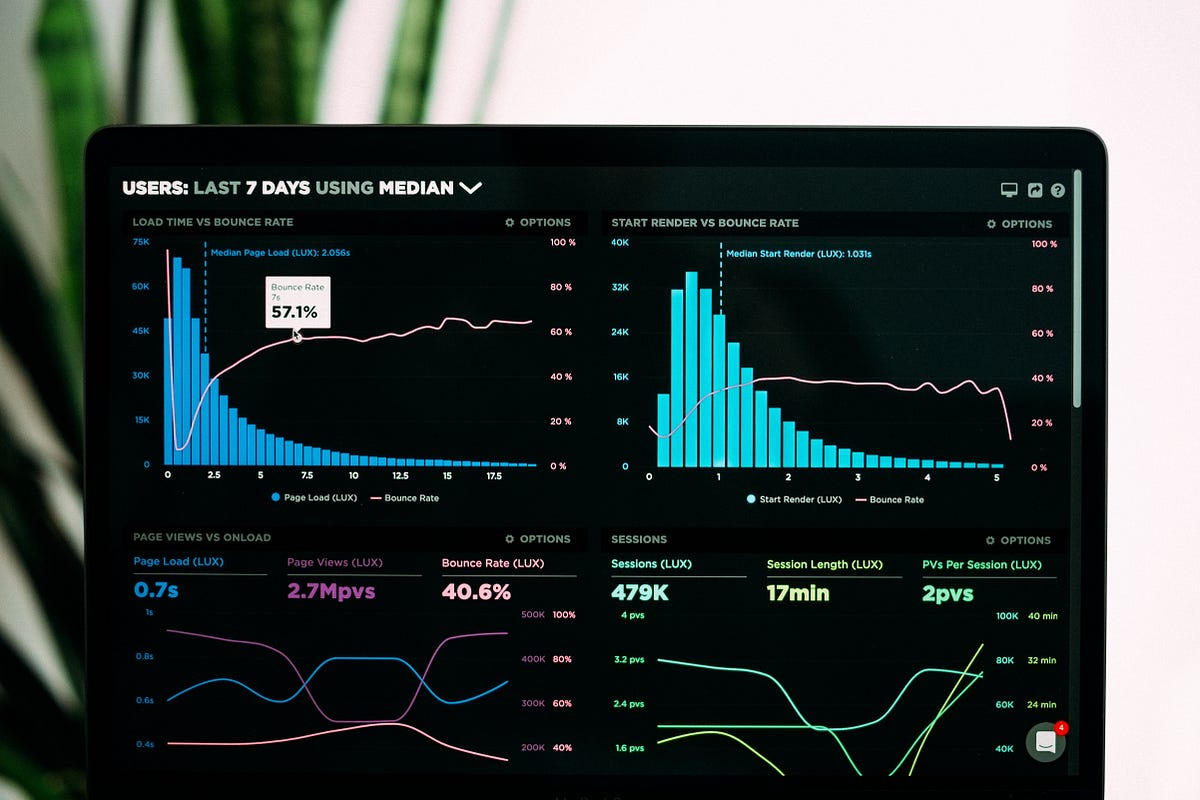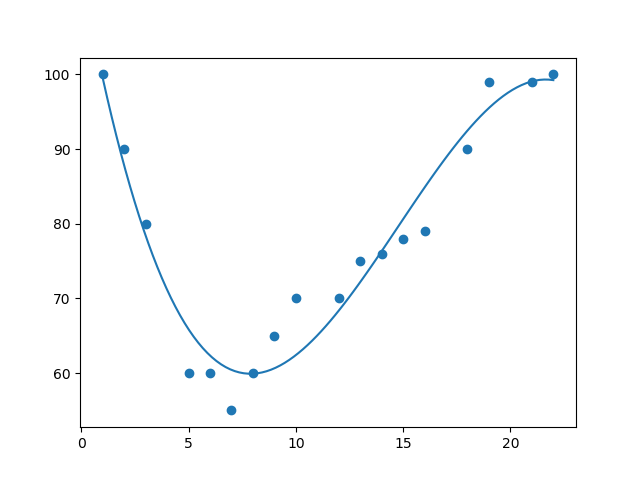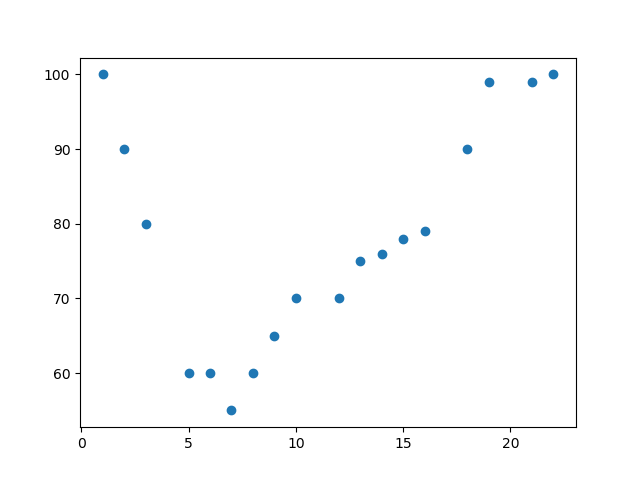# The Ultimate Beginners Guide to Polynomial Regression in Python.If your data points clearly will not fit a linear regression it might be ideal for polynomial regression. Machine Learning is making the computer learn from studying data and statistics. Machine Learning is a step into the direction of artificial intelligence (AI). Machine Learning is a program that analyses data and learns to predict the outcome.

Machine Learning is making the computer learn from studying data and statistics. Machine Learning is a step into the direction of artificial intelligence (AI). Machine Learning is a program that analyses data and learns to predict the outcome.

## What is Regression?

The term regression is used when you try to find the relationship between variables. In Machine Learning and statistical modelling, that relationship is used to predict the outcome of future events.

Polynomial Regression

Polynomial regression, like linear regression, uses the relationship between the variables x and y to find the best way to draw a line through the data points.Python has methods for finding a relationship between data-points and to draw a line of polynomial regression. We will show you how to use these methods instead of going through the mathematic formula. In the example below, we have registered 18 cars as they were passing a certain tollbooth.

The x-axis represents the hours of the day and the y-axis represents the speed:

``````import matplotlib.pyplot as plt

x = [1,2,3,5,6,7,8,9,10,12,13,14,15,16,18,19,21,22]
y = [100,90,80,60,60,55,60,65,70,70,75,76,78,79,90,99,99,100]

plt.scatter(x, y)
plt.show()``````

Result## Data Science Projects | Data Science | Machine Learning | Python

Practice your skills in Data Science with Python, by learning and then trying all these hands-on, interactive projects, that I have posted for you.

## Data Science Projects | Data Science | Machine Learning | Python

Practice your skills in Data Science with Python, by learning and then trying all these hands-on, interactive projects, that I have posted for you.

## Data Science Projects | Data Science | Machine Learning | Python

Practice your skills in Data Science with Python, by learning and then trying all these hands-on, interactive projects, that I have posted for you.

## Data Science Projects | Data Science | Machine Learning | Python

Practice your skills in Data Science with Python, by learning and then trying all these hands-on, interactive projects, that I have posted for you.

## Data Science Projects | Data Science | Machine Learning | Python

Practice your skills in Data Science with Python, by learning and then trying all these hands-on, interactive projects, that I have posted for you.